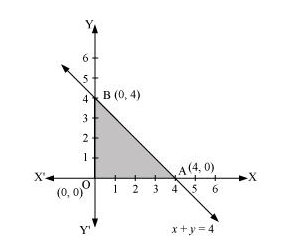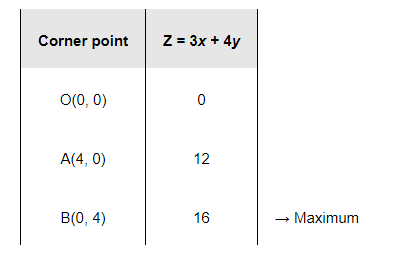# Maximise

Question:

Maximise Z = 3x + 4y

Subject to the constraints: $x+y \leq 4, x \geq 0, y \geq 0$.

Solution:

The feasible region determined by the constraints, $x+y \leq 4, x \geq 0, y \geq 0$, is as follows.The corner points of the feasible region are O (0, 0), A (4, 0), and B (0, 4). The values of Z at these points are as follows.Therefore, the maximum value of Z is 16 at the point B (0, 4).